### DTRANSPOSEDdata science, AI, technology Blog About Projects

Research Paper Summary - The AI Economist: Improving Equality and Productivity with AI-Driven Tax Policies | DTRANSPOSED

# Research Paper Summary - The AI Economist: Improving Equality and Productivity with AI-Driven Tax PoliciesAll the figures in this post come from the research publication: The AI Economist: Improving Equality and Productivity with AI-Driven Tax Policies (Zheng et al. 2020)

It is really refreshing to see that machine learning, especially reinforcement learning, can be successfully used in social sciences. The recent research from the Salesforce team, the AI Economist, is a study on how to improve economic design through the AI-driven simulation. The goal of the study is to optimise productivity and social equality of the economy. The AI framework has been designed to simulate millions of years of economies to help economists, governments and others design tax policies that strive for fair social outcomes in the real world.

I am personally really excited about the fact, that we can use simulation and reinforcement learning to replicate the mechanics of economic behaviour and generate data, which is otherwise very expensive and difficult to collect. It takes decades or even centuries to generate information about economic activity of some economies. So how about, instead of waiting that long, replicating the behaviour of the population using fast, parallel simulated worlds?

There are two types of actors in the economic simulation: AI Citizens (members of the community focused on maximising their wealth) and the AI Government (the overseer which attempts to maximise the social welfare of the community).

## The AI Citizens

### Gather-and-Build Game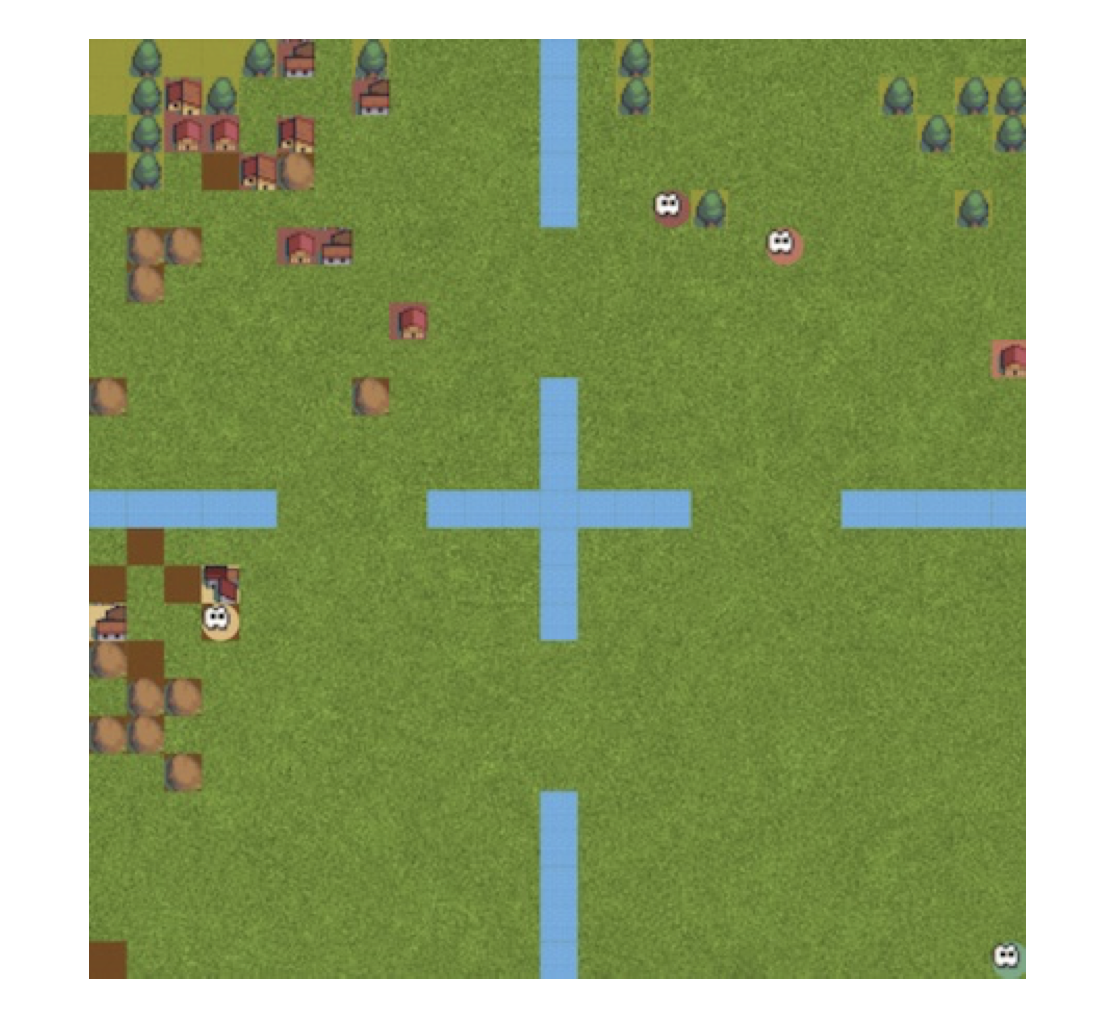The rules of the modelled world, the Gather-and-Build Game, are quite simple. One could even argue that the simplicity of the simulation is the one of the biggest flaws of the study. Obviously, the hand-designed environments are bound to miss many of the subtleties of economics.

The community consists of four citizens. Each of the citizens can choose between four actions:

• Move - up, down, left, right in the 2d grid-world environment.
• Harvest resources - there are two assets in the game, wood and stone.
• Trade - there is an in-game trade market implemented, where agents can publish bids and ask prices to buy or sell the resources.
• Build houses - to build a house, an agent needs to spend one unit of wood and one unit of stone.

Additionally, every action requires some labor to be performed by the agent. This is quite an important feature of agent’s psychology: doing any kind of work is inherently undesirable by the rational agent.

### Heterogeneities

Heterogeneities of the citizens are the main driver of inequality and specialisation in the simulation. Agents differ in:

• Spawn location - if an agent spawns close to the “forest”, it is more likely that it will specialise in collecting and trading wood.
• Builder skill - high builder skill means that building houses is more profitable for an agent.
• Harvester skill - high harvester skill means that an agent has high probability of gaining bonus resources when collecting wood or stone.

### Citizen’s Goal

Citizens are fundamentally simplistic creatures. Their goal is to become wealthy. However, not at all costs. They also strive to avoid performing any labor at all. How? In economics and AI, the desires or preferences of rational agents are being modelled by the utility function. If we consume some kind of item, we derive satisfaction (utility) from it. Our citizen $$i$$ at time $$t$$ derives pleasure from its coins $$x_{i,t}^{c}$$ and dissatisfaction from performing labor $$l_{i,t}$$:

$u_i(x_{i,t},l_{i,t})=crra(x^{c}_{i,t})-l_{i,t}$

Let’s take a look at the $$crra$$ function. It is agreed that the utility of homo economicus (rational, economic human) is not linear - it is governed by the law of diminishing returns. The law states that the marginal utility of a good declines as its available supply increases. This can be illustrated by the example.

Imagine it’s a hot, summer day and you lucky to be participate in all-you-can eat ice-cream buffet. You have an unlimited supply of the treat, so you start consuming ice-cream, one after another. Let’s assume that the utility for eating the ice-cream is initially equal to one:

• Your first ice-cream will be cold, refreshing and very delicious (marginal utility of 1).
• Your third ice-cream will still be sweet and tasty, but not as amazing as the first one (marginal utility is smaller, let’s say 0.5).
• You will probably refuse to eat the tenth ice-cream - otherwise you will get quite queasy (negative utility - you don’t even want to any more ice-cream!)

This law is implemented in our agents behaviour through the function $$crra$$. In the context of our simulation, we can substitute ice-cream with houses. We can see that the utility grows initially with the amount of houses built by the agent, but at some point it starts to decline.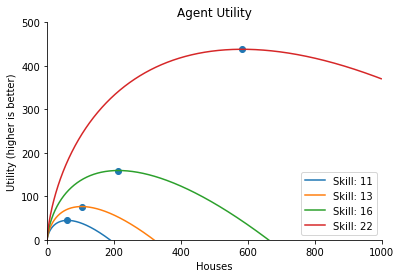The goal of the citizens is to maximum the sum of their total discounted future utility:

$\forall i: \max_{\pi_i} \mathbb{E}_{ a_i \sim \pi_i, \mathbf{a}_{-i} \sim \mathbf{\pi}_{-i}, s' \sim \mathscr{T} }[{ \sum_{t = 1}^H \gamma^t \underbrace{({ u_i(x_{i,t}, l_{i,t} ) - u_i(x_{i,t-1}, l_{i,t-1} ) }}_{=\hspace{2pt} r_{i,t}} + u_i( x_{i,0}, l_{i,0} )] }$

### Example of an EpisodeThe figure above show the economic simulation in which four agents collect and trade resources, build houses and earn income. Red and orange agents were endowed with very low house-building skill, so they specialise in collecting and trading wood/stones for coins. The teal agent seems to be the jack of all trades, building moderate amounts of houses and collecting some resources. Dark blue agent (high house-building skill) actively buys most of the resources from the market and floods the world with its houses.

The size of the circle shows total productivity, while colours of the pie chart show the percentage of economy’s wealth each agent owns. The trade-off between equality and productivity is measured by multiplying equality and productivity.

## The AI Government

The AI Government is the entity which oversees the community and tries to come up with a tax policy which is the most beneficial for the overall economy.

### Collecting Taxes

Every episode is divided into ten periods. At the end of each period, the government observes citizen’s income (total number of coins) - $$z^{p}_{i}$$ of an agent $$i$$ after the period $$p$$ - and takes away some of its capital governed by the tax function $$T$$ . Finally, once all taxes are collected, the government sums them up and redistributes the amount equally among the community.

So the post-tax income of agent $$i$$ in the period $$p$$ is given by:

$\widetilde{z}^p_i = z^p_i - T(z^p_i) + \frac{1}{N} \sum_{j=1}^N T(z^p_j).$

The amount of tax $$T(z)$$ imposed on agent’s income $$z$$ in a tax period $$p$$ is computed by taking the sum of the amount of income within each bracket $$[m_b, m_{b+1}]$$ times that bracket’s marginal rate $$\tau_b$$:

$T(z)=\sum_{b=0}^{B-1} \tau_b \cdot ({ ({m_{b+1} - m_b })\mathbf{1}[z > m_{b+1} ] + ({z - m_b }) \mathbf{1}[m_b < z \leq m_{b+1} ] }$

where $$\mathbf{1}[ z > m_{b+1}]$$ is an indicator function for whether $$z$$ saturates bracket $$b$$ and $$\mathbf{1}[ m_b < z \leq m_{b+1} ]$$ is an indicator function for whether $$z$$ falls within bracket $$b$$.

### AI Government’s Goal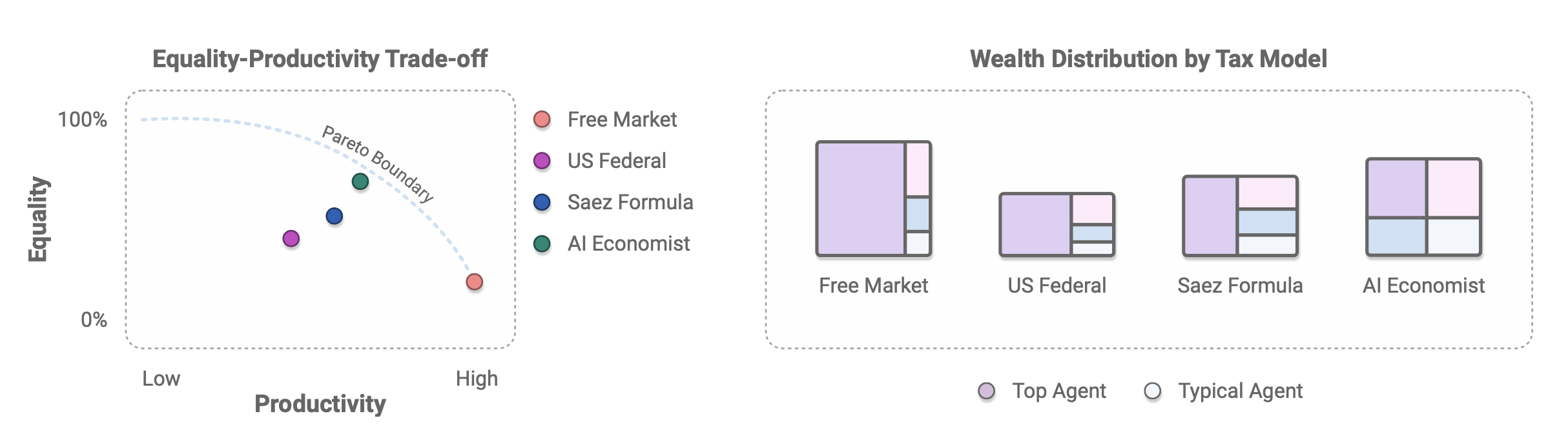The AI government’s goal is to maximise the social warfare of the community, defined by the social welfare function $$swf$$. The social welfare function can be defined in many ways, but in this paper the authors decide to tackle the fundamental trade-off between income equality and productivity.

• If income equality is high, this means that the most productive members of the community are financially supporting the least productive agents (which is characteristic for e.g. centrally planned economies). Obviously, those most productive members are disincentivized - they do not want to perform labor from which they do not gain any coins - so the productivity falls.

• If productivity is high (essentially unregulated, free market), the most productive members thrive, but agents who are less fortunate (e.g. are “born” with low skills) barely make a living.World map of the GINI coefficients by country. Based on World Bank data ranging from 1992 to 2018. Source: wikipedia

The economic quantity which gauges of economic inequality is the Gini index. Therefore, to express the inequality we can use the compliment of the Gini index computed for the toy community.

$eq(\mathbf{x}^c)=1 - gini(\mathbf{x}^c)\frac{N}{N-1}$

To measure the economic productivity, we can take the sum of all the coins in the economy:

$prod(\mathbf{x}^c)=\sum_{i=1}^{N}x_i^c$

The social welfare function is simply the product of income equality and productivity:

$swf_t(\mathbf{x}_t^c) = eq_t(\mathbf{x}_t^c)\cdot prod_t(\mathbf{x}_t^c)$

The AI Government’s (denoted as $$p$$) objective is to maximise the social welfare:

$\max_{\pi_p} \mathbb{E}_{ tau \sim \pi_p, \mathbf{a}_{i} \sim \mathbf{\pi}_{i}, s' \sim \mathscr{T} }[{ \sum_{t = 1}^H \gamma^t \underbrace{({ swf_t -swtf_{t+1}) }}_{=\hspace{2pt} r_{p,t}} + swf_0] }$

## Two-Phase Training

The authors report that the joint optimisation of the AI citizens and AI Government is not straightforward and may suffer from training instability in early episodes (which is not surprising giving that the joint objective resembles a min-max game). To solve this problem, the two-phase training approach is being used:

• Phase 1: agents are being trained in the tax-free world (essentially free-market scenario).
• Phase 2: the training is continued, but community is gently introduced to the AI Government and the concept of income redistribution. To avoid unstable learning dynamics created by the sudden introduction of taxes, the marginal tax rates are linearly annealed from 10% to 100%.

## The AI Taxation Policy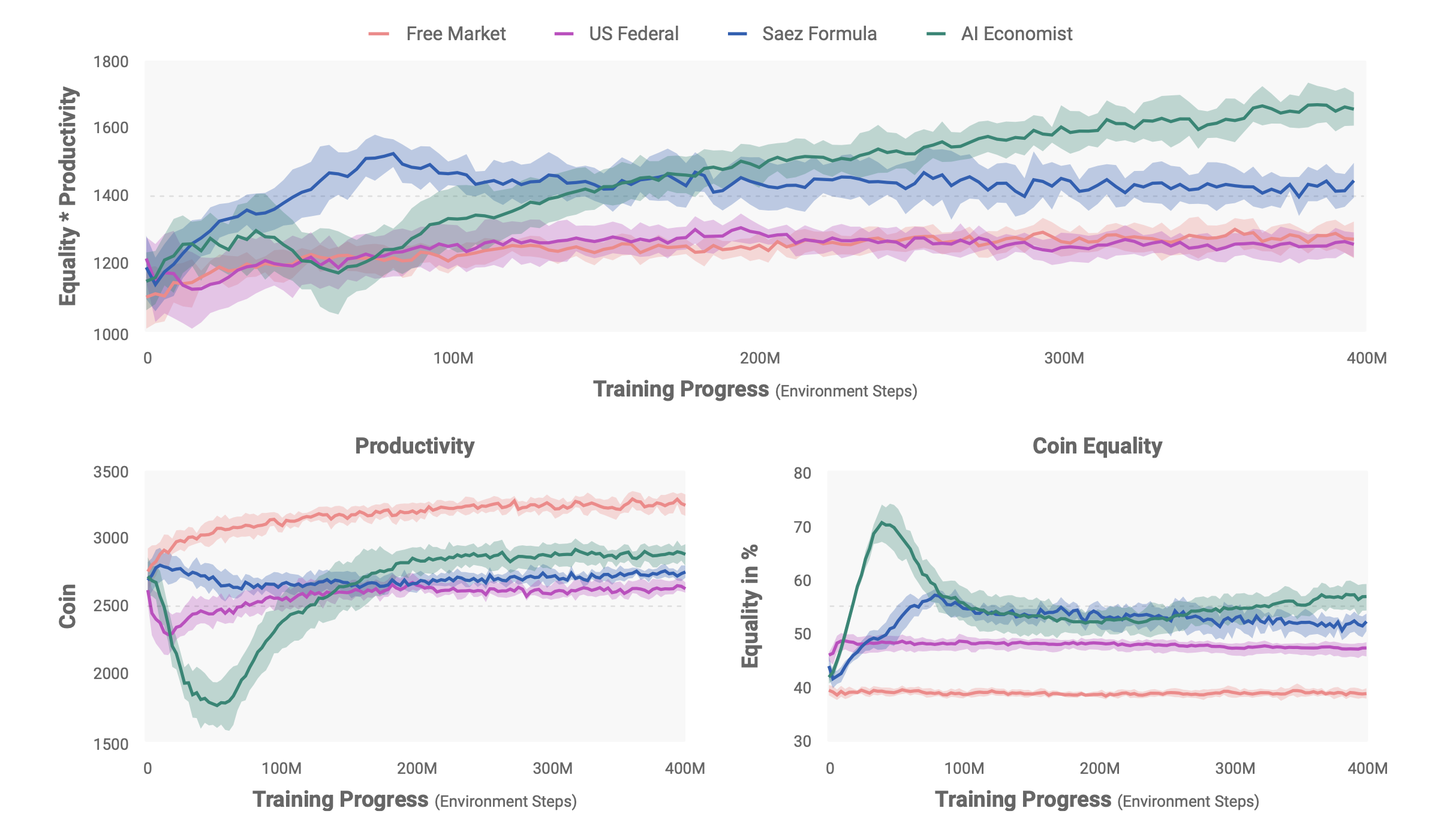The figure illustrates the comparison of overall economic outcomes. The AI Economist achieves significantly better equality-productivity trade-offs compared to the baseline models: free-market economy, US-Fed tax policy and Saez formula (model of optimal income tax rate developed by Emmanuel Saez (2001). Note, that the AI Economist, while initially being very socialistic (prefers equality to productivity), finally converges to the optimal equilibrium point, where those two objectives are relatively balanced.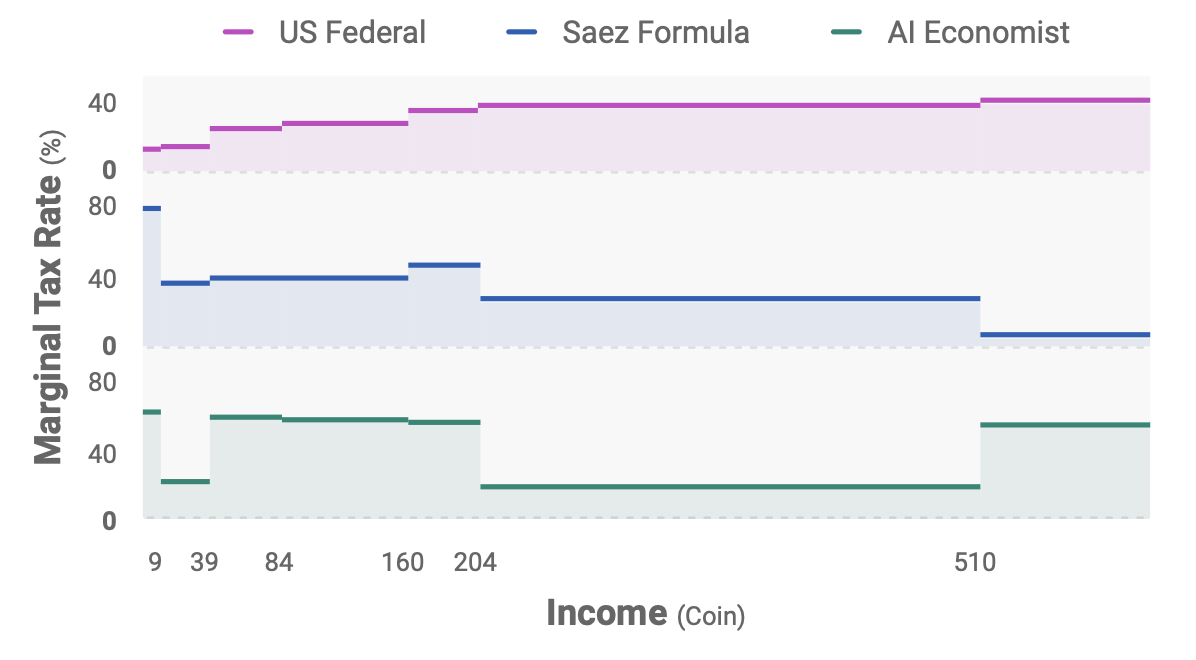The figure shows the marginal taxes rates for each of the baselines (except for the free-market where taxes do not exist). On average, the AI Economist sets a higher top tax rate than both of the US Federal and Saez tax schedules. It seems that it favours two groups of citizens: agents which earn little (but no too little) and the “middle-class”. Those two groups benefit from sharply reduced tax rates. Maybe the AI gives us a hint, that we should keep the taxes low for the middle class? Or that we shall tax the poorest to incentivise them to work?

Truth be told, I am quite sceptical about saying that the AI Government has “came up” with some certain taxation policy - the model is far too simple. After all, the simulated economy consists of only four citizens and it seems that every episode results in the same occupation distribution. Those are two low-income agents (stone and wood harvesters), one “jack of all trades” and one high-income house builder: not really a very robust result.

### Applying AI Taxation to Real Humans

The researchers conclude the paper with an interesting study. They employ human participants on Amazon Mechanical Turk platform to investigate whether AI Economist tax policy can transfer to economic activity of real people without extensive fine-tuning. Human participants play the Gather-and-Build Game with the goal of maximising their wealth, while being the subject to baseline and AI Economist taxation policies.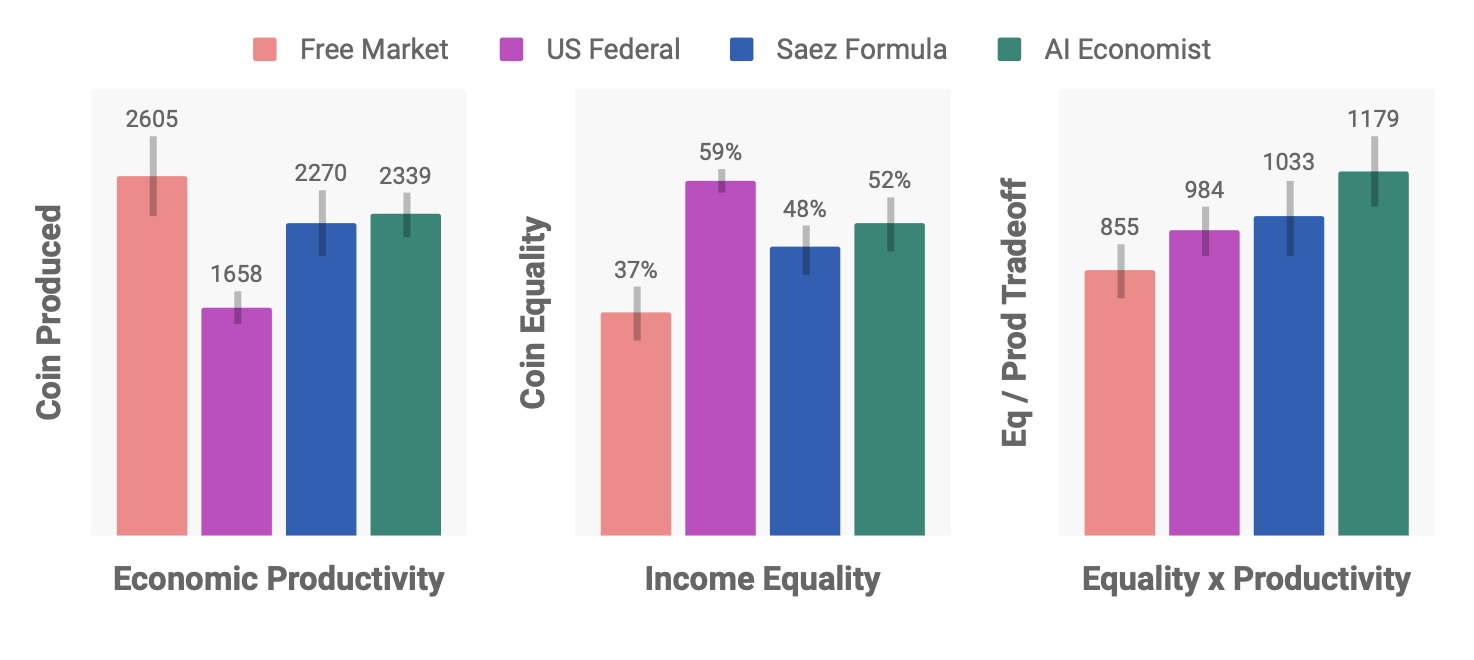The figure above presents the results of tax policy transfer for game with 58 human participants in 51 episodes. Each episode involves four participants. The AI Economist achieves competitive equality-productivity trade-offs with the baselines, and statistically significantly outperforms the free market.

What really sparks my interest is the fact, that the behaviour of human participants and trained AI agents differ significantly. For example, it has been observed that humans tend to block other players and otherwise display adversarial behaviour.

## Conclusion

This work from Salesforce team shows that AI-based, economic simulators for learning economic policies have the potential to be useful in the real world. I am really excited to see more research, which tries to apply AI to social sciences and solve some of the vast puzzles, which we encounter in economy both in the micro and macro scale. For more information, check out the paper, as well as the Salesforce’s blog post on the AI Economist. They also published a great video intro to the publication on YouTube. Finally, the project’s code has been released to the public!